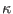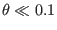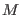# 6 Conclusions

In this work, we have investigated nonlinear characteristics of electrostatic solitary wave structures in a collisionless plasma consisting of adiabatic cool electrons, mobile cool positrons (electron holes), hot-distributed electrons and immobile ions. We have derived a linear dispersion relation, and studied the effects of positron parameters on the dispersion characteristics, through the positron-to-cool electron density ratio. It is found that the phase speed increases weakly with an increase in(see Fig. 1). Similarly, in agreement with the previous finding , increasing suprathermality (decreasing) significantly reduces the phase speed.

The Sagdeev's pseudopotential technique was used to determine nonlinear structures and the range of allowed Mach numbers of electrostatic solitons. The results of this study indicate that increasing the positron-to-cool electron density ratioand the positron-to-hot electron temperature ratiolead to a slightly narrowing of the Mach number range for negative polarity solitons (Fig. 2). Moreover, the upper Mach number limit for positive polarity solitons slightly decreases with increasingand decreasing(Fig. 3). However, the lower Mach number limit for positive polarity solitons is found to be at aboutin the parameter ranges of the positrons (and). From Fig. 3 one can see that increasingtoward a Maxwellian distribution increases the upper limit of Mach numbers for positive polarity solitons.

The e-p model predicts the existence of positive potential solitons associated with the positrons, in addition to negative potential solitons. It is found that increasing the positron-to-cool electron density ratiodecreases the normalized negative potential (Fig. 4), and increases the normalized positive potential (Fig. 5) in the ranges of allowed Mach numbers for negative and positive polarity solitons, respectively. The disturbances and amplitudes of cool electron density and cool electron velocity due to the solitary waves decrease with increasing, as well as the disturbances and amplitudes of positron density and velocity decrease in the negative polarity electrostatic mode (Fig. 4). However, higherincreases and steepens the normalized positive potential, the disturbances and amplitudes of positron density and velocity, and cool electron density and velocity in the positive polarity electrostatic mode (Fig. 5). Therefore, increasing the positron density increases the electric potential amplitude in the positive polarity electrostatic mode, whereas decreases it in the negative polarity electrostatic (electron-acoustic) mode.

We also note that at fixed values of the normalized soliton speed,, the amplitudes of the perturbations of positron density and velocity are significantly increased and steepened with higher values of the positron-to-hot electron temperature ratio(Fig. 6). As the positrons constitute a small fraction of the total number density (), the normalized potential, cool electron density and velocity are trivially affected by. Therefore, thermal effects of the cool positrons are negligible for both negative and positive electric potentials.

From Fig. 7, it can be seen that the suprathermality can significantly raise the electric potential amplitude in the positive polarity electrostatic mode. This means that suprathermal electrons play a key role in rising a positive potential pulse from a tiny fraction of cool positrons. Therefore, we expect to have a strong positive polarity electrostatic wave when the suprathermality is stronger (lower). Fig. 8 demonstrates how the pulse amplitude of positive polarity electrostatic solitons rises with reducing the spectral index(higher suprathermality) at a fixed true Mach number (), while the soliton amplitude increases with the true Mach number for all values of.

In conclusion, the results of this study suggest that the dynamics of electrostatic solitary waves can be modified by a small fraction of cool positrons (or electron holes) in the presence of suprathermal electrons. The results of this study could have important implications for positive polarity electrostatic waves observed in the auroral magnetosphere [34,35], as well as the formation of coherent radio emission in pulsars [41,42,43,44,45], where positrons and suprathermal electrons are present.

Ashkbiz Danehkar
2018-03-28